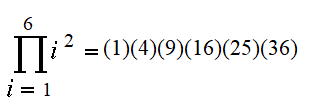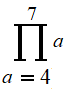# Product Notation (Pi Notation)

Product notation (also called pi notation) indicates repeated multiplication.

For example, the following product notation represents the product of the first six squared numbers (from i = 1 to 6):This notation is very similar to summation notation. The only difference is that while summation notation expresses a pattern of sums (i.e. a sequence of numbers), product notation shows a pattern in products. Instead of the Greek letter sigma (Σ), we use the upper case Greek letter pi (Π).

We use product notation to prevent the tedium of writing out long lists of products; While three dots “…” can also work as a shorthand (and often does!), sometimes it doesn’t work if the pattern isn’t very clear .

## Example Questions

Example question #1: Write out the following product:Solution:

Step 1: Locate the starting point at the base of the Π:For this example, that’s a = 4. In other words, we’re going to start multiplying at “4”:Step 2: Locate the ending point at the top of the Π. For this example, we’re going to stop multiplying at the number 7, so:Example question #2: Write out the following product:This time, the products are defined for elements of a set of four numbers. This particular variation on the notation is telling us to multiply the number “3” for every element in the set.

Solution:## Formal Definition of Product Notation

The formal definition is stated as :

If m and n are integers with m ≤ n, then the symbol(which reads as “the product from k equals n to n of a-sub-k”), is the product of all of the terms am, am + 1, am + 2, … an.

## References

 Tsun, A. Chapter 0. Prerequisites. 0.3: Sum and Product Notation. Retrieved April 21, 2021 from: https://courses.cs.washington.edu/courses/cse312/20su/files/student_drive/0.3.pdf
 Chapter 5: Sequences, Mathematical Induction, and Recursion. Retrieved April 21, 2021 from: https://people.ohio.edu/melkonia/math3050/HW/Section5.1.pdf

CITE THIS AS:
Stephanie Glen. "Product Notation (Pi Notation)" From StatisticsHowTo.com: Elementary Statistics for the rest of us! https://www.statisticshowto.com/product-notation-pi-notation/